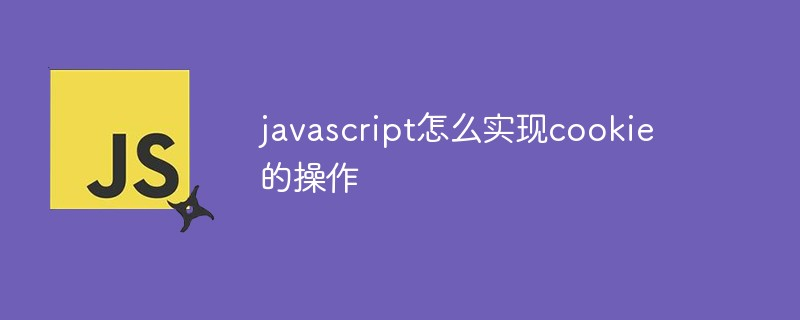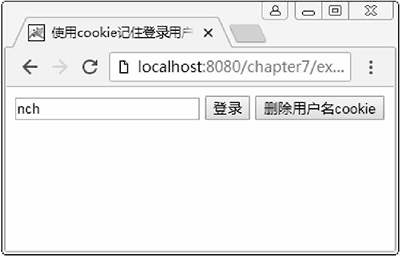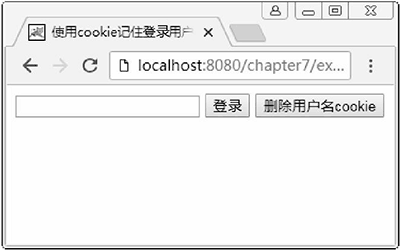`document.cookie = "名称1=值1[; 名称2=值2; …]";`

```document.cookie = "username=abc";

```document.cookie = "username=abc; age=23";
for(var i = 0; i < arr1.length; i++){
var arr2 = arr1[i].split('=');
if(arr2 == 'age'){
}
}```

`document.cookie = "名称=值;expires="+字符串格式的时间;`

```var oDate = new Date();
oDate.setDate(oDate.getDate()+10);//访问页面后的10天过期

```var oDate = new Date();
oDate.setDate(oDate.getDate()-1);//访问页面的前一天

```<!doctype html>
<html>
<meta charset="utf-8">
<script>
var oDel = document.getElementById('del');
//判断用户是否曾经登录过
}
for(var i = 0; i < arr1.length; i++){
var arr2 = arr1[i].split('=');
if(arr2 == key){
return unescape(arr2);//对编码后的内容进行解码
}
}
}
var oDate = new Date();
oDate.setDate(oDate.getDate()+t);
//使用escape()对内容进行编码
}
}
}
oDel.onclick = function(){
}
};
</script>
<body>
</body>
</html>```【推荐学习：javascript高级教程